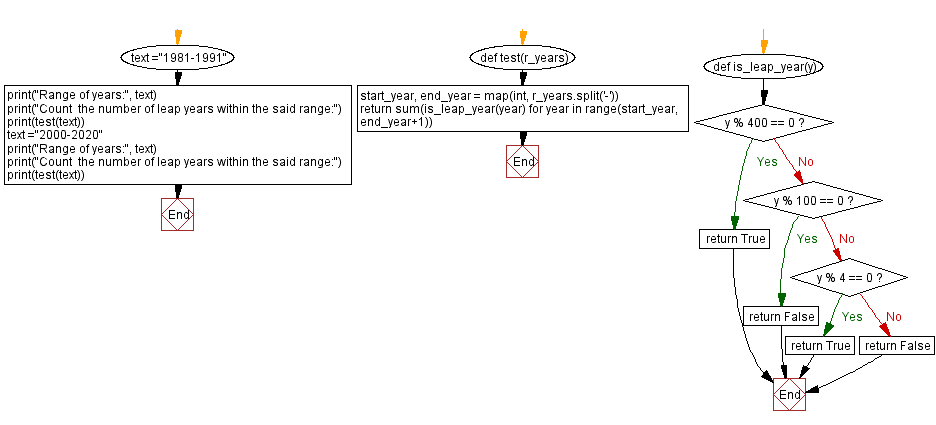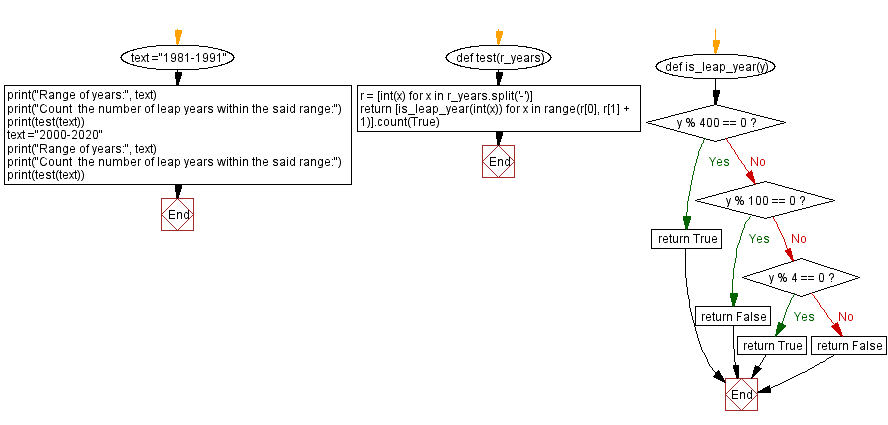﻿ Python - Count the number of leap years within the range# Python Exercises: Count the number of leap years within the range

## Python String: Exercise-109 with Solution

Write a Python program that counts the number of leap years within the range of years. The range of years should be accepted as a string.

Sample Data:
("1981-1991") -> 2
("2000-2020") -> 6

Sample Solution-1:

Python Code:

``````def test(r_years):
start_year, end_year = map(int, r_years.split('-'))
return sum(is_leap_year(year) for year in range(start_year, end_year+1))
def is_leap_year(y):
if y % 400 == 0:
return True
if y % 100 == 0:
return False
if y % 4 == 0:
return True
else:
return False

text ="1981-1991"
print("Range of years:", text)
print("Count  the number of leap years within the said range:")
print(test(text))
text ="2000-2020"
print("Range of years:", text)
print("Count  the number of leap years within the said range:")
print(test(text))
```
```

Sample Output:

```Range of years: 1981-1991
Count  the number of leap years within the said range:
2
Range of years: 2000-2020
Count  the number of leap years within the said range:
6
```

Flowchart:## Visualize Python code execution:

The following tool visualize what the computer is doing step-by-step as it executes the said program:

Sample Solution-2:

Python Code:

``````def test(r_years):
r = [int(x) for x in r_years.split('-')]
return [is_leap_year(int(x)) for x in range(r, r + 1)].count(True)

def is_leap_year(y):
if y % 400 == 0:
return True
if y % 100 == 0:
return False
if y % 4 == 0:
return True
else:
return False

text ="1981-1991"
print("Range of years:", text)
print("Count  the number of leap years within the said range:")
print(test(text))
text ="2000-2020"
print("Range of years:", text)
print("Count  the number of leap years within the said range:")
print(test(text))
```
```

Sample Output:

```Range of years: 1981-1991
Count  the number of leap years within the said range:
2
Range of years: 2000-2020
Count  the number of leap years within the said range:
6
```

Flowchart:## Visualize Python code execution:

The following tool visualize what the computer is doing step-by-step as it executes the said program:

Python Code Editor:

Have another way to solve this solution? Contribute your code (and comments) through Disqus.

Previous Python Exercise: Hash elements.
Next Python Exercise: Insert space before capital letters in word.

What is the difficulty level of this exercise?

Test your Programming skills with w3resource's quiz.

﻿

## Python: Tips of the Day

Getting sorted iterable (can sort by "compare" function):

```>>> a = [1, 2, -3]
>>> sorted(a)
[-3, 1, 2]

>>> sorted(a,key=abs)
[1, 2, -3]
```